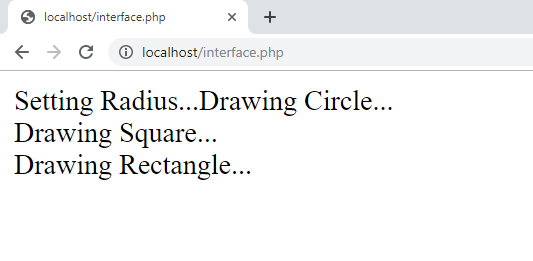Open in App
Not now

# What is the point of interfaces in PHP ?

• Last Updated : 24 Oct, 2019

An Interface allows users to create programs specifying the public methods that a class must implement, without involving the complexities and details of how the particular methods are implemented. It is generally referred to as the next level of abstraction. It resembles the abstract methods, resembling the abstract classes. An Interface is defined just like a class is defined but with the class keyword replaced by the interface keyword and the function prototypes. The interface contains no data variables. The interface is helpful in a way that it ensures to maintain a sort of metadata for all the methods a programmer.
In PHP, we can do Object-Oriented Programming. That means we can make use of Object-Oriented concepts like create classes and objects, use inheritance, etc. OOPs contains mainly three different types of inheritance. Which are: Single Inheritance, Multiple Inheritance, and Multi-level Inheritance. Now, PHP supports single and multi-level inheritance. Multiple inheritance is not directly supported in PHP but can be implemented using Interface. So, the main reason for Interfaces in PHP is to implementing Multiple Inheritance.
In multiple inheritance, we can derive a child class from two or more parent classes. Here is how we can implement multiple inheritance in PHP.

We have three different classes as follows:

```class Circle {
public function draw(){
//
}
//
}
}

class Square {
public function draw(){
//
}
}

class Rectangle {
public function draw(){
//
}
}
```

Now as it is shown above, we have the same function in all the three classes draw() and one more function in the class Circle as setRadius(). Now we have three different interfaces created and implemented them in our classes as follows:

```interface Shape {
public function draw();
}

}

interface Main {
public function process();
}

class Circle implements Shape, Radius, Main {
public function draw(){
echo "Drawing Circle...";
}
}
public function process() {
\$this->draw();
}
}

class Square implements Shape, Main {
public function draw(){
echo "Drawing Square...";
}
public function process() {
\$this->draw();
}
}

class Rectangle implements Shape, Main {
public function draw(){
echo "Drawing Rectangle...";
}
public function process() {
\$this->draw();
}
}
```

As it is shown above, we have implemented more that one interface in our classes. The Shape and Main interfaces are implemented in all the classes but Radius interface is just implemented in Circle class.

Then a fourth class called DrawShape is there as we want to call the process() function in all the classes. The process() function is to call all the functions in that class.

```class DrawShape {
public function newShape(Main \$shape) {
return \$shape->process();
}
}
```

Example:

 `"``;``    ``}`` ` `    ``public` `function` `setRadius() {``        ``echo` `"Setting Radius..."``;``    ``}`` ` `    ``// To call all the functions in this class``    ``public` `function` `process() {``        ``\$this``->setRadius();``        ``\$this``->draw();``    ``}`` ` `}`` ` `class` `Square ``implements` `Shape, Main {`` ` `    ``public` `function` `draw(){``        ``echo` `"Drawing Square..."``;``        ``echo` `"
"``;``    ``}`` ` `    ``// To call all the functions in this class``    ``public` `function` `process() {``        ``\$this``->draw();``    ``}`` ` `}`` ` `class` `Rectangle ``implements` `Shape, Main {`` ` `    ``public` `function` `draw(){``        ``echo` `"Drawing Rectangle..."``;``        ``echo` `"
"``;``    ``}`` ` `    ``// To call all the functions in this class``    ``public` `function` `process() {``        ``\$this``->draw();``    ``}`` ` `}`` ` `class` `DrawShape {`` ` `    ``public` `function` `newShape(Main ``\$shape``) {``        ``return` `\$shape``->process();``    ``}``     ` `}`` ` `// To Draw Circle``\$shapeCircle` `= ``new` `Circle();``\$drawCircle` `= ``new` `DrawShape();``\$drawCircle``->newShape(``\$shapeCircle``);`` ` `// To Draw Square``\$shapeSquare` `= ``new` `Square();``\$drawSquare` `= ``new` `DrawShape();``\$drawSquare``->newShape(``\$shapeSquare``);`` ` `// To Draw Rectangle``\$shapeRectangle` `= ``new` `Rectangle();``\$drawSquare` `= ``new` `DrawShape();``\$drawSquare``->newShape(``\$shapeRectangle``);`` ` `?>`

Output:My Personal Notes arrow_drop_up